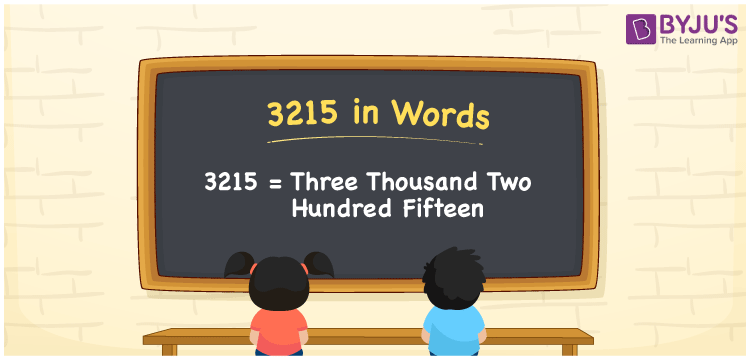# 3215 in Words

3215 in English is written as Three Thousand Two Hundred Fifteen. For instance, Sonal purchased a saree worth Rs. 3215, then you can say, “Sonal purchased a saree worth Rupees Three Thousand Two Hundred Fifteen”. The English alphabet is used to convert numbers to words. The number 3215 is a cardinal number since it shows the value of something. Learning the numbers in word form help students to understand and express the numbers in a better way.

 3215 in Words Three Thousand Two Hundred Fifteen Three Thousand Two Hundred Fifteen in numerical form 3215

## 3215 in English Words

Generally, numbers in words are represented using the English alphabet. Hence, we can express the number 3215 in English as Three Thousand Two Hundred Fifteen.## How to Write 3215 in Words?

We use a place value chart for easy conversion of numbers to words. Here, 3215 is a four-digit number. Hence, let us make a table of 4 columns as mentioned below.

 Thousand Hundreds Tens Ones 3 2 1 5

Therefore, we can write the expanded form as:

3 x Thousand + 2 x Hundred + 1 x Ten + 5 x One

= 3 x 1000 + 2 x 100 + 1 x 10 + 5 x 1

= 3000 + 200 + 10 + 5

= 3215

= Three Thousand Two Hundred Fifteen

Hence, 3215 in words is written as Three Thousand Two Hundred Fifteen

Interesting way of writing 3215 in words

3 = Three

32 = Thirty-Two

321 = Three Hundred and Twenty-One

3215 = Three Thousand Two Hundred Fifteen

Thus, the word form of the number 3215 is Three Thousand Two Hundred Fifteen

3215 is a natural number that is one less than 3216 and one greater than 3214

• 3215 in words – Three Thousand Two Hundred Fifteen
• Is 3215 an odd number? – Yes
• Is 3215 an even number? – No
• Is 3215 a perfect square number? – No
• Is 3215 a perfect cube number? – No
• Is 3215 a prime number? – No
• Is 3215 a composite number? – Yes

## Frequently Asked Questions on 3215 in Words

Q1

### How do you write 3215 in words?

3215 in words is written as Three Thousand Two Hundred Fifteen.
Q2

### Simplify 2000 + 1215, and express in words.

Simplifying 2000 + 1215, we get 3215. Thus, 3215 in words is Three Thousand Two Hundred Fifteen.
Q3

### Write Three Thousand Two Hundred Fifteen in numbers.

Three Thousand Two Hundred Fifteen in numbers is 3215.